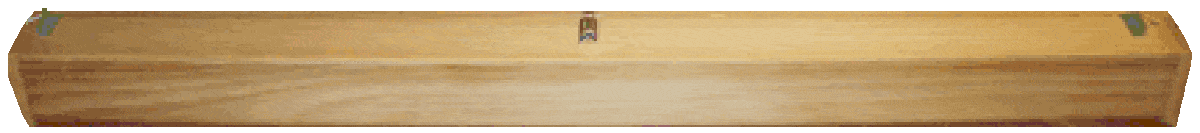# Finding Intervals on the Monochord

posted in: Music History & Library | 0## Finding Intervals on the Monochord

Two Methods:

There are different ways to find intervals on the monochord. One way is to divide the string by 1/2,1/3,1/4,1/5, etc., thus giving the harmonic series.
This way is quite straightforward. The second way is to divide the string by the mathematical proportion itself. For instance a string divided into three parts and two parts would give the fifth, a ratio of 3:2.

We are explaining here the second of the two ways for two reasons. The first reason is that when finding an interval by dividing the string by a half, third, fourth, fifth, sixth and so on, you must always go back to the open string. This could slightly change the tuning of the open string because the center bridge would have to be removed to hear the open string. The second reason is that the intervals go out of the octave of the open string, into higher octaves and could be more difficult for your students to hear.

Tuning by the proportions found among the Greeks

All the bridges on your monochord are moveable. The ratio of the octave is 2:1, the fifth 3:2, the fourth 4:3, the major third 5:4, the minor third 6:5, the major sixth 5:3, the minor sixth 8:5, the whole tone 9:8.

The Octave-ratio 2:1

Place the right bridge on 99 and the center bridge on 66.The string is now
divided in a ratio of 2:1 (66:33). Have your students play the string on either side of the middle bridge to hear the octave.

___________________________________66_________________________99

The fifth-ratio 3:2
Here we are dealing with a ratio which has 5 parts to it (3+2). 5 goes into 100, our complete ruler 20 times (100 divided by 5 equals 20). 20 times 3 equals 60 and 20 times 2 equals 40. Place the middle bridge on 60. The entire string should be on 100. Play both sides of the string the interval is the fifth.

__________________________________________60_____________________________100

The interval of the fourth-ratio 4:3

The numbers 4 and 3 have 7 parts (4+3). Divide 100 by 7 and you get 14 plus a remainder. We won't use the remainder but will multiply 7 times 14 to get 98 (the closest number to 100). 4 times 14 is 56 and 3 times 14 is 42.Move the right bridge to 98 and place the center bridge on 56. your students will hear the interval of the fourth.

_____________________________________56__________________________________98

Major third 5:4

Place the middle bridge on 55, the entire length is 99.

_______________________________________55__________________________________99

Minor third 6:5 ratio

Place the middle bridge on 54. the entire string length is 99. The interval heard on either side of the middle bridge is the minor third.

______________________________________54__________________________________99

The major sixth 5:3 ratio
Place the middle bridge on 60. The entire length of the string should be 96.

________________________________________60_________________________________96

The minor sixth-8:5 ratio

Place the middle bridge on 56. The entire string length should be 91. Play both sides of the string, the interval is the minor sixth.

______________________________________56__________________________________91

The whole tone, ratio 9:8

Place the middle bridge on 45. The entire string should be 85.

___________________________________45_____________________________________85

The half step was called a limma by the Greeks. To find this interval the entire ruler should be 99.8, while the middle bridge is 51.2. The ratio of this interval is 256:243. The Greeks even have another type of half tone, the apotome which has a ratio of 2187:2048. This ratio can also be found on the monochord. However if you think the Greeks went a little too far with their proportions, they didn't come close to our equal temperament (tuning of the piano) a ratio of 1,059,463,094 : 1 ,000,000,000, the ratio of our half tone

Back to the Teacher's Room